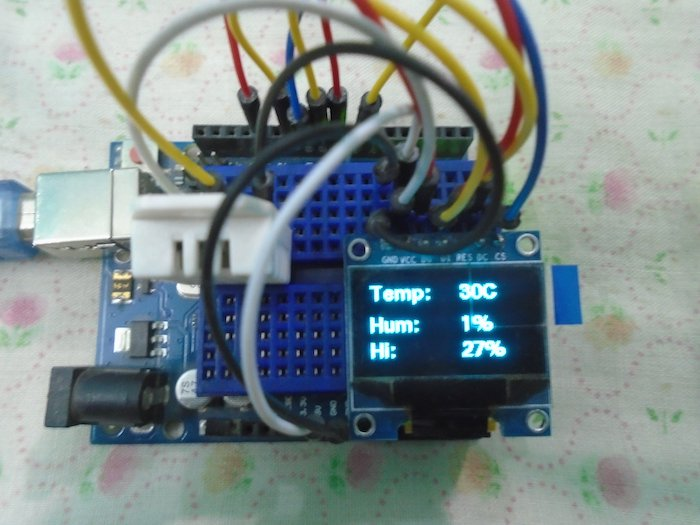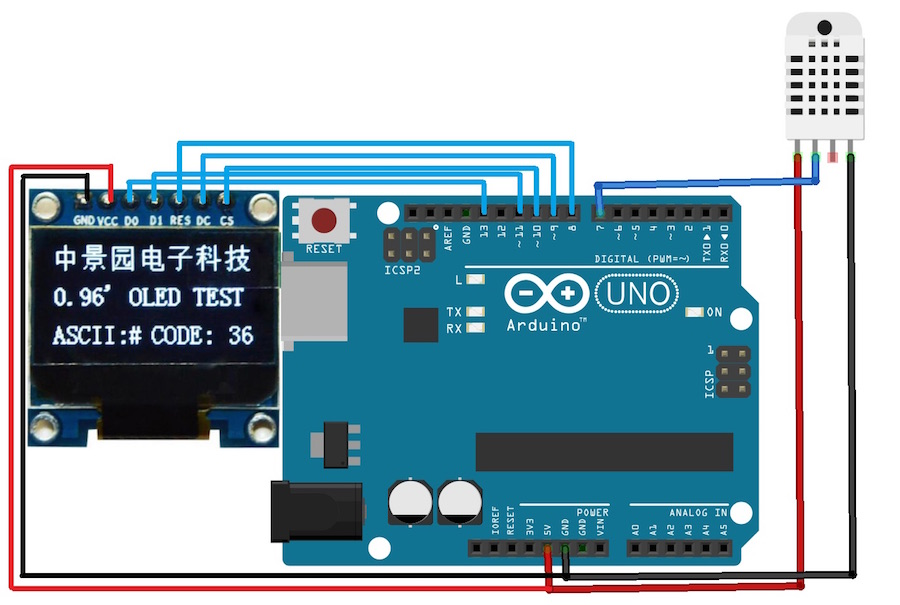This Arduino thermometer and hygrometer takes temperature and humidity readings and displays the data on an OLED screen.

In this project, we are going to make an Arduino OLED thermometer and hygrometer. We will read the temperature and humidity from the DHT22 sensor and then we will show the data on the OLED screen.

OLED stands for organic light emitting diode and they are available in many different sizes. The size we are going to use is 128X64 (1.3’’). The OLED works with the Arduino through SPI as well as I2C communication but in our project, we are going to use the SPI communication.### Circuit Diagram

First of all, we will connect the OLED with the Arduino. The OLED can be connected to the Arduino in I2C as well as SPI. The connections for connecting the OLED in the I2C way are easier but SPI communication is faster than the I2C. So, we are going to connect the OLED with the Arduino using SPI. Make the connections of the OLED with the Arduino as follows:

• Connect the CS pin on the OLED to pin 10 on the Arduino
• Connect the DC pin on the OLED to pin 9 on the Arduino
• Connect the RST pin on the OLED to pin 8 on the Arduino
• Connect the D1 or CLK pin on the OLED to pin 11 on the Arduino
• Connect the D0 or DIN pin on the OLED to pin 13 on the Arduino
We have connected the OLED to pins 13, 11, 10, 9, and 8 because these pins are for SPI communication. Next, connect the DHT22 with the Arduino. The connections for DHT22 sensor with Arduino are as follows:

• Connect the VCC on DHT22 to the 5V pin on the Arduino
• Connect the GND on the DHT22 to the GND on the Arduino
• Connect the data pin of the DHT22 to pin 7 on the Arduino### Arduino Code

```#include
#include "DHT.h"
#define DHTPIN 7
#define DHTTYPE DHT22
DHT sensor(DHTPIN, DHTTYPE);

U8GLIB_SH1106_128X64 oled (13, 11, 10, 9, 8);

void setup()
{
sensor.begin();
oled.firstPage();
do
{
oled.setFont(u8g_font_fur14);   	//setting the font size
//Printing the data on the OLED
oled.drawStr(20, 15, "Welcome");
oled.drawStr(40, 40, "To");
oled.drawStr(5, 60, "DIYHACKING");
}  while( oled.nextPage() );
delay(5000);
}

void loop()
{
if (isnan(h) || isnan(t) || isnan(fah)) {		//Checking if we are receiving the values or not
Serial.println("Failed to read from DHT sensor!");
return;
}
float heat_index = sensor.computeHeatIndex(fah, h);	//Calculating the heat index in Fahrenheit
float heat_indexC = sensor.convertFtoC(heat_index);    //Calculating the heat index in Celsius

oled.firstPage();
do
{
oled.setFont(u8g_font_fub11);   	//setting the font size
//Printing the data on the OLED
oled.drawStr(0, 15, "Temp: ");
oled.drawStr(0, 40, "Hum: ");
oled.drawStr(0, 60, "Hi: ");
oled.setPrintPos(72, 15);     		//setting the dimensions to print the temperature
oled.print(t, 0);
oled.println("C");
oled.setPrintPos(72, 40);		//setting the dimensions to print the humidity
oled.print(h, 0);
oled.println("%");
oled.setPrintPos(72, 60);		//setting the dimensions to print the heat index
oled.print(heat_indexC, 0);
oled.println("%");
}
while( oled.nextPage() );
delay(2000);
}

```

### Code Explanation

First, we include the library for the DHT22 sensor and for the OLED. The ‘U8glib’ library is for the OLED and it makes the code very easy. We will show the data on the OLED by using the functions of ‘U8glib’ library.

```#include
#include "DHT.h"
```
Next, we defined the pin where we have connected the DHT22 sensor data pin and then defined the type of DHT sensor. There are some other types of DHT sensors available in the market. After that, we initialized the pins where we have connected the OLED.

```#define DHTPIN 7
#define DHTTYPE DHT22
DHT sensor(DHTPIN, DHTTYPE);
U8GLIB_SH1106_128X64 oled(13, 11, 10, 9, 8);
```
In the setup function, we give the command to start receiving the values from the DHT22 sensor. Then we set the font and printed “Welcome to DIYHACKING” on the OLED for 5 seconds. You can change the font size if you don’t like that. You can find different font sizes here.

```sensor.begin();
oled.firstPage();
do
{
oled.setFont(u8g_font_fur14);   	//setting the font size
//Printing the data on the OLED
oled.drawStr(20, 15, "Welcome");
oled.drawStr(40, 40, "To");
oled.drawStr(5, 60, "DIYHACKING");
}  while( oled.nextPage() );
delay(5000);
}
```
In the loop function, we read the values of the humidity and the temperature from the DHT22 sensor and then we calculated the heat index using the temperature and the humidity.

```float h = sensor.readHumidity(); 		//Reading the humidity value
if (isnan(h) || isnan(t) || isnan(fah)) {		//Checking if we are receiving the values or not
Serial.println("Failed to read from DHT sensor!");
return;
float heat_index = sensor.computeHeatIndex(fah, h);	//Calculating the heat index in Fahrenheit
float heat_indexC = sensor.convertFtoC(heat_index);    //Calculating the heat index in Celsius
```
Lastly, we again set the font size and printed the temperature, humidity, and heat index on the OLED. You can change the font size by following the link which is discussed above and also you can set the data at different dimensions.

```oled.firstPage();
do
{
oled.setFont(u8g_font_fub11);   	//setting the font size
//Printing the data on the OLED
oled.drawStr(0, 15, "Temp: ");
oled.drawStr(0, 40, "Hum: ");
oled.drawStr(0, 60, "Hi: ");
oled.setPrintPos(72, 15);     		//setting the dimensions to print the temperature
oled.print(t, 0);
oled.println("C");
oled.setPrintPos(72, 40);		//setting the dimensions to print the humidity
oled.print(h, 0);
oled.println("%");
oled.setPrintPos(72, 60);		//setting the dimensions to print the heat index
oled.print(heat_indexC, 0);
oled.println("%");
}
while( oled.nextPage() );
delay(2000);
}
```